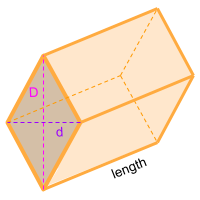Home » Polyhedra » Prisms » Rhombic Prism

# Volume of a Rhombic PrismVolume =
D × d/2
× Length

## Volume calculator for a rhombic prism

Enter the Long Diagonal (D) and the Short Diagonal (d) of the rhombus that forms one of the bases of the prism along the length of the rhombic prism to get the volume automatically.

## Description, how many faces, edges and vertices are there in a rhombic prism

The rhombic prism, is a shape that if a cross section is made in any part of its length, always maintains the shape of a rhombus. It has 6 faces, 2 of which are rhombus and form the bases at the ends of the solid, these bases are parallel, it has 12 edges and 8 vertices.

## Formula for the volume of a rhombic prism

To calculate the volume of a rhombic prism you can use the formula to calculate the volume of all prisms. where the area of one of the bases is multiplied by the length of the prism. In this case, the base of the rhombic prism is a rhombus, therefore the area of the rhombus needs to be a known value. To get the area of a rhombus, the long and short diagonal need to be multiplied and then divided by 2, finally multiply the result by the length of the prism. You can also use the online calculator to calculate the volume of the diamond prism automatically.

Volume =
D × d/2
× Length

Formula explanation:

The formula to calculate the volume of prism is always the same:

Volume prism = Area base × Length

In this case, the area of the base of the rhombic prism is a rhombus:

Area base = Area rhombus =
D × d /2

Replacing the calculated area in the formula for volume of prisms we get the formula shown above.

Share